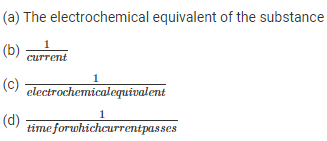# Current Electricity MCQ for NEET

NEET  Physics is the scoring paper in the medical entrance examination. Here, you will discover the NEET Physics MCQ Questions for all Concepts as per the latest syllabus. Practice more on a regular basis with these NEET Physics objective questions on air pollution and improve your subject knowledge & problem-solving skills along with time management. NEET Physics Current Electricity Multiple Choice Questions make you feel confident in answering the question in the exam & increases your scores to high.

## MCQ on Current Electricity

1. What does the internal resistance of a cell mean?
(a) The resistance of the material used in the cell
(b) The vessel of the cell
(c) The electrodes of the cell
(d) The electrolyte used in the cell

Answer: (d) The electrolyte used in the cell

2. A potentiometer is considered a versatile and accurate device to make an electrical measurement of EMF. Why?
(a) Because the method involves a combination
(b) There are cells involved
(d) As there is no flow of current through the galvanometer

Answer: (d) As there is no flow of current through the galvanometer

3. Consider copper and aluminium wire whose length and resistance are the same. Also, the specific resistance of aluminium is more than copper. Select the correct statement
(a) Copper and aluminium mass are the same
(b) Copper wire has more mass than the aluminium wire
(c) Copper wire has less mass than the aluminium wire
(d) The information given is incomplete

Answer: (d) The information given is incomplete

4. Following is the graph which represents the thermo EMF of two different metals which are joined end to end. One end of the metal is kept at a constant temperature while the other end is heated at very high temperature.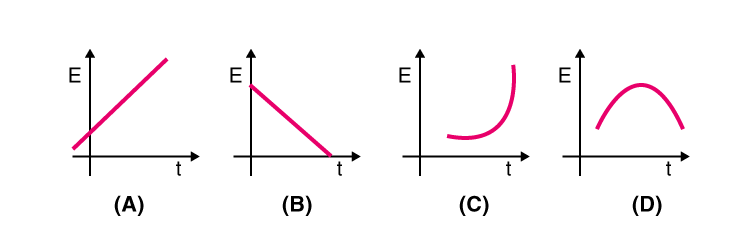5. Select the graph which depicts the variation in thermoelectric power and the temperature difference between the hot and cold junction in the thermocouples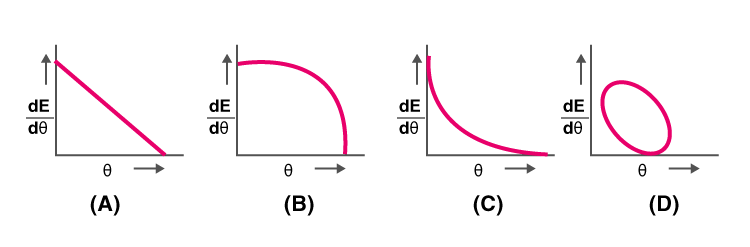6. Which of the following represents the relationship between the current flowing, i through the electric heater and the time taken, t.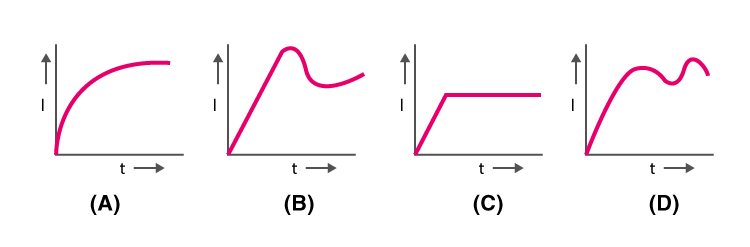7. Consider three voltmeters A, B, and C with resistance R, 1.5R, and 3R respectively. Potential difference is applied across the points X and Y, the voltmeter readings are VA, VB, and VC.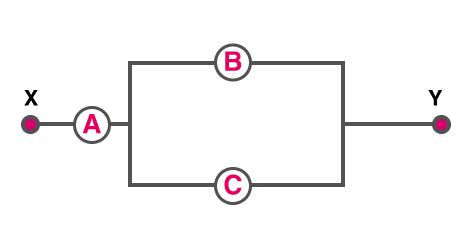(a) VA = V≠ VC
(b) VA ≠ V≠ VC
(c) VA = V= VC
(d) VA ≠ V= VC

Answer: (c) VA = V= VC

8. What is the ratio of power dissipated if two electric bulbs are connected in series and the ratio of their resistance is 1:2?
(a) 1:4
(b) 1:2
(c) 2:1
(d) 1: 1

9. Following is the Wheatstone bridge in which $$\frac{P}{Q}=\frac{R}{S}$$. Let K be the key which is closed, so what will be the deflection shown by galvanometer?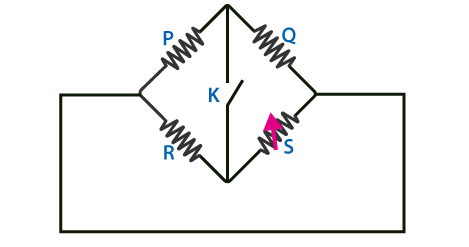(a) The deflection happens in either side
(b) There is no deflection
(c) Deflection happens on the right side
(d) Deflection happens on the left side

Answer: (a) The deflection happens in either side

10. What is the principle behind thermocouple
(a) Joule effect
(b) Thomson effect
(c) Seebeck effect
(d) Peltier effect